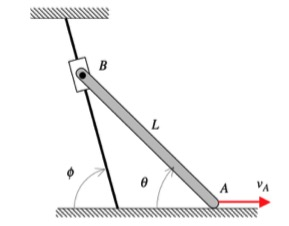# Homework H2.F - Sp23

 Problem statement Solution video https://youtu.be/lHCWDbNV9a4DISCUSSION and HINTS

In this problem, end A of the bar is constrained to move along a straight horizontal path with a constant speed of vA, whereas end B is constrained to move along a straight, angled path. As you can see in the animation below of the motion of the bar, the speed of B is NOT a constant (the acceleration of B is non-zero, and is, in fact, increasing as B moves along its path).

In your solution, it is recommended that you use the rigid body kinematics equations relating the motion of ends A and B:

vB = vA + ω x rB/A
aB = aA + α x rB/A - ω2rB/A

For these equations, you know: i) the magnitude and direction for the velocity of A; ii) that the acceleration of A is zero (constant speed along a straight path); and, iii) the direction for the velocity and acceleration of B. These two vector equations produce four scalar equations that can be solved for four scalar unknowns: vB, aB, ω and α.## 17 thoughts on “Homework H2.F - Sp23”

1.Alicja Stoppel says:

For this problem, I set up the velocity equations for B with respect to A then with respect to O, which is the point on the ground where the angle is 45. I did this because we needed another equation since there's too many unknowns for one equation. However, in the end you technically get a value for the angular velocity of the rigid body that O is on (the line that B slides on), but isn't it supposed to be zero since it's stationary? I know this answer doesn't ask to solve for it but you'll need it later on when solving for acceleration in the same method...unless this method is incorrect, in which case I'm confused on how to solve for the angular terms.

1.Bernardo de Barros Barreto C Machado says:

I dont think were solving for the angular terms, they're just being used as a variable that you solve for to use in the equations for the normal velocity and acceleration

1.Alicja Stoppel says:

But it doesn't say to find the answer in terms of the angular velocity/acceleration, which is why I thought we should solve for them

2.James Jung says:

How do we find the position vector for B?

1.Alicja Stoppel says:

I drew a vertical line down from B so that it's perpendicular to the ground. From there, you'll have to use trig, the angles, and the L of the bar to solve for the x and y components of B

1.CMK says:

Alicja's response is right on for the position of B relative to A, r_B/A, as need for the kinematics equations.

2.Lily Waterman says:

You can set up two triangles using the given angles phi and theta and length L and use trig and hypotenuse theorem to solve for the i and j components. It is helpful to note that since phi = 45º, two of the sides are the same length.

3.Andrew Thomas Zaslavsky says:

I used the phi angle for B's position vector. We know from the hints that the velocity and acceleration are going down across the wire. One component of the velocity would be Vb * cos (phi) for example.

3.Michael Thomas Beisner says:

Given the angle phi as equal to 45 degrees, the i and j components for Vb should be equal to each other, same for Ab. that gives you four equations to find four unknowns.

1.Michael Thomas Beisner says:

*The magnitudes of the i and j components should be equal, the signs are determined from the diagram

4.Michael Thomas Beisner says:

I'm pretty sure you cannot relate B to a fixed point other than A, because B is moving and they are not a fixed distance apart. The equations are only valid for points on the same rigid body.

5.Luis Martinez says:

When I first read through the problem I had no idea how the angle phi was going to be used. Like Micheal pointed out above in this case the magnitudes of the i and j components of Vb are equal, but I think it is important to note that if we were given a different angle such as 60 degrees we would still simply be able to write Vb in component form using trig and keeping our equations equal to our unknowns in that way.

6.Landon J Vuagniaux says:

My initial thought was to use phi for the position vector of B, but I realized theta must be used because the position vector is obviously with respect to A. Phi is used later on when balancing the i and j components of Vb. Sin(phi) and cos(phi) are equivalent, so the i and j components can be set equal, which will lead us to finding one of our unknowns.

7.Salma Alrowaie says:

If the velocity of v_b is constant in a specific direction, wouldn't a_b be 0? Unless I made a calculation error

1.CMK says:

That would be true if v_B were constant. It is given that v_A is constant; however, that does not say that v_B = constant. Therefore, a_B is probably not zero.

8.Rachel Elizabeth Rizzi says:

I'am still confused in what format the answers should be in. Since the question doesn't say to solve in terms of vectors does that mean to solve for the exact value or it doesn't matter?

1.CMK says:

Since velocity and acceleration are vectors, you should provide your final answers as vectors. Cartesian components would make sense.